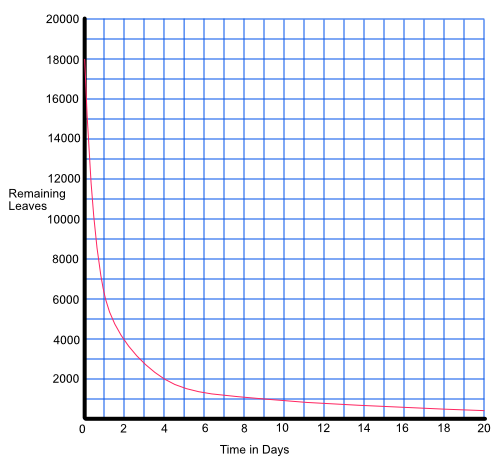Plot and Interpret Reciprocal Graphs

# Plot and Interpret Reciprocal Graphs

GCSE(F), GCSE(H),

To plot a reciprocal graph, use several points. A reciprocal graph will have two lines, and both lines will be curved and tend toward the asymptotes. In real-life situations, only the positive part (above the x-axis) of the graph is shown. In algebraic usage, the negative part, which will be partially or totally below the y-axis, may also be shown.

Reciprocal graphs tend to show efficiency (fuel used against speed, the number of people allocated to a task against the time taken to complete it), or growth/decay (half-life of a radioactive sample).

## Examples

1. A tree loses its leaves in inverse proportion to time: early in Autumn it loses many per day; later in Autumn it loses only a few per day. The function can be modelled using: frac(s)(d + 1), where s is the number of leaves on the tree at the start of Autumn and d is the number of days into the season.

Plot the graph for 20 days into the season, where the initial number of leaves was 18 000.

Answer:2. Using the graph in question 1, estimate how many leaves will still be on the tree after 9 days.